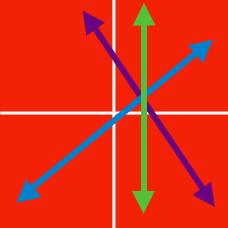Algebra

# System of Linear Equations - Problem Solving

Find the sum of all values of constant $k$ such that there exists non-zero solutions (i.e $x \neq 0$ and $y \neq 0$) to the following equations: $4x+y=kx, \;\;\; 12x+15y=ky.$

Let $\text{Max}(x, y)$ and $\text{Min}(x, y)$ be defined as follows for real numbers $x$ and $y:$ $\text{Max}(x, y)=\begin{cases} x \quad (x\ge y) \\ y \quad (x

If the following holds for distinct numbers $x$ and $y,$ what is $3xy:$ $\text{Max}(x, y)=5x-2y+79, \quad \text{Min}(x, y)=4x+3y-47?$

Consider the system of linear equations \begin{aligned} 3x+Ay &= -108 \ \mbox{and} \\ -\frac{x}{2}+\frac{y}{3} &= B, \end{aligned} where $A$ and $B$ are constants. If this system has an infinite number of solutions, what is the value of $B-A$?

Let $x=a$ and $y=b$ be the solution of the simultaneous equations $\frac{5x-3}{12}+\frac{7y+6}{8}=1$ and $\frac{(x+53)}{D} = \frac{2y}{6}$ If $b=a+5$, what is the value of the constant $D$?

If $x=A$ and $y=B$ are the solutions of the simultaneous equations $\frac{3y-4x+16}{2}=\frac{3x-y}{3}=2x-y,$ what is the value of $A+B$?

×